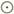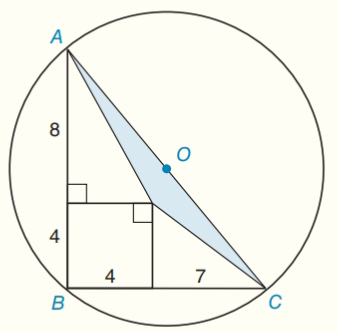Chapter 8.CR, Problem 17CR### Elementary Geometry for College St...

6th Edition
Daniel C. Alexander + 1 other
ISBN: 9781285195698

#### Solutions

Chapter
Section### Elementary Geometry for College St...

6th Edition
Daniel C. Alexander + 1 other
ISBN: 9781285195698
Textbook Problem
11 views

# If A C ¯ is a diameter ofO, find the area of the shaded triangle.Exercise 17

To determine

To find:

The area of the shaded triangle.

Explanation

Theorem:

1. The area A of a triangle whose base has length b and whose corresponding altitude has length h is given by

A=12bh

2. The area A of a square whose sides are each of length s is given by A=s2

Calculation:

Sketch the following diagram:

To find ΔAABC:

ΔAABC=12bh=12×11×12=11×6=66 cm2

To find ABFDE:

ABFDE=s2=42=16cm2

To find ΔACDF:

### Still sussing out bartleby?

Check out a sample textbook solution.

See a sample solution

#### The Solution to Your Study Problems

Bartleby provides explanations to thousands of textbook problems written by our experts, many with advanced degrees!

Get Started

#### In Exercises 5-10, solve for y in terms of x. 4x+y=7

Calculus: An Applied Approach (MindTap Course List)

#### In Exercises 1124, find the indicated limits, if they exist. 21. limxx2x21

Applied Calculus for the Managerial, Life, and Social Sciences: A Brief Approach

#### The third partial sum of is:

Study Guide for Stewart's Multivariable Calculus, 8th

#### True or False: is a convergent series.

Study Guide for Stewart's Single Variable Calculus: Early Transcendentals, 8th